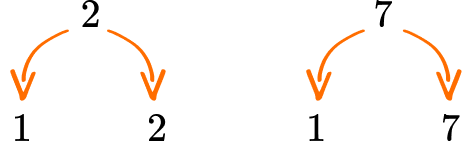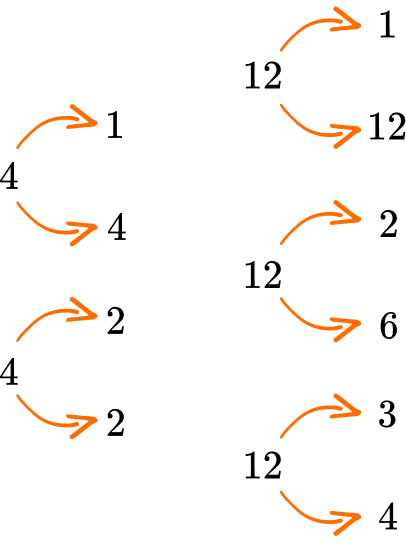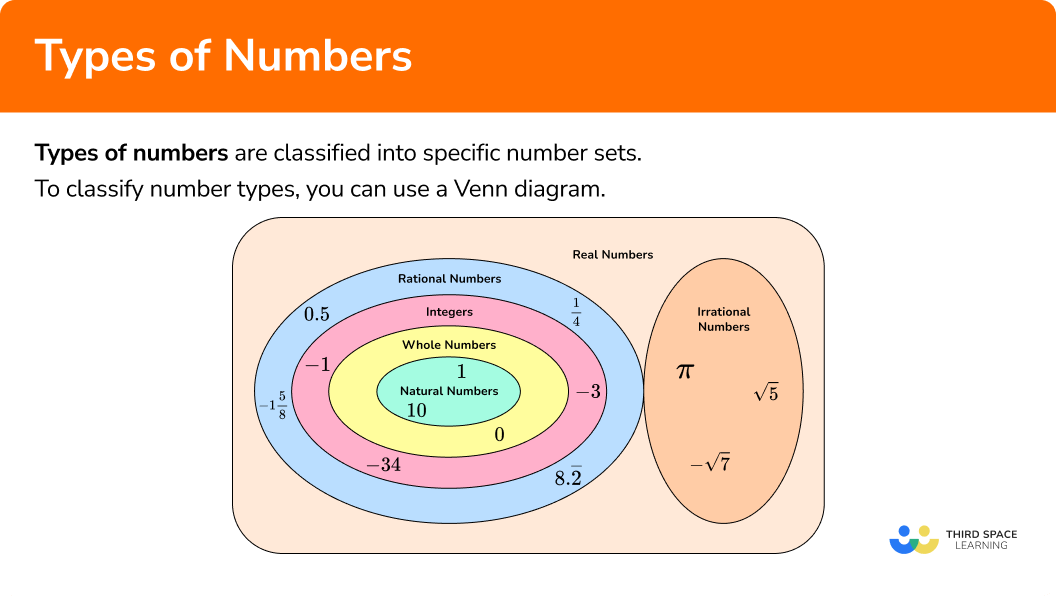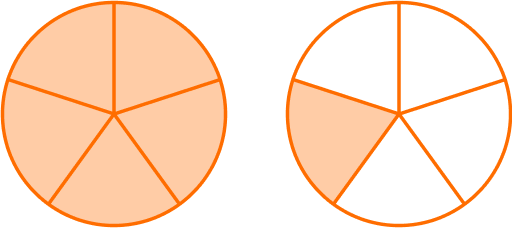# Types of numbers

Here you will learn about different types of numbers and how they are related and classified.

Students begin to learn about types of numbers beginning in kindergarten and develop that knowledge from elementary school through high school.

## What are types of numbers?

Types of numbers are classified into specific number sets. In elementary school, you will work with the set of rational numbers.

Let’s look at the different types of numbers and how they are classified.

Number SetsDescriptionSamples

Natural Numbers

Positive whole numbers also
known as the counting numbers.

1, 2, 3, 4, 5, 6, ...

Whole Numbers

Positive whole number plus 0.

0, 1, 2, 3, 4, 5, 6 ...

Integers

Positive and negative whole
numbers, plus 0.

…-3, -2, -2, 0, 1, 2, 3...

Rational Numbers

Any number that can be expressed
as a fraction where the numerator
and denominator are integers. This
includes positive and negative whole
numbers, terminating decimals, and
repeating decimals.

-5, 0, 1, \cfrac{4}{5} \, , 1\cfrac{3}{4} \, , -1.\overline{2}, 9.5, 0.\overline{3}

Irrational Numbers

A number that cannot be expressed
as a fraction. This includes positive
and negative non-repeating,
non-terminating decimals.

\sqrt{5}, -\sqrt{11}, \sqrt{2}, \pi

Real Numbers

All of the rational numbers plus the
irrational numbers.

1, \cfrac{4}{5} \, , \sqrt{12}, 0.\overline{53}, 0.\overline{12}, -7, 0, 34

Special classifications

Prime and composite numbers

A prime number is a number with exactly two factors: itself and 1. 2 and 7 are examples of prime numbers.2 has 2 factors: 7 has 2 factors:
1, 2 \hspace{2.5cm} 1, 7

1 \times 2 = 2 \hspace{2cm} 1 \times 7 = 7

A composite number is a number with more than two factors. 4 and 12 are examples of composite numbers4 has 3 factors: 12 has 6 factors:
\hspace{.4cm}1, 2, 4 \hspace{1.9cm} 1, 2, 3, 4, 6, 12

\hspace{.2cm}1 \times 4 = 4 \hspace{1.7cm} 1 \times 12 = 12
2 \times 2 = 4 \hspace{1.7cm} 2 \times 6 = 12
\hspace{3cm} 3 \times 4 = 12

Even and Odd Numbers

Even numbers are divisible by 2 without remainders; they end in 0, 2, 4, 6, or 8.

Odd numbers are not divisible by 2 without remainders and end in 1, 3, 5, 7, or 9.

Positive and negative numbers

Positive numbers are numbers that are greater than 0. On the number line, they are to the right of 0. Positive numbers can be whole numbers, fractions, or decimals.
Negative numbers are numbers that are less than 0. On the number line, they are to the left of 0. Negative numbers can be whole numbers, fractions, or decimals.

Fractions

Fractions are numbers that are equal parts of a whole. They have a numerator and a denominator separated by a fraction bar.

Fractions can also be interpreted as division.

Numerator → dividend

Denominator → divisor

Fraction bar → division bar

Types of fractions:

• Proper fractions are fractions where the numerator is less than the denominator.
For example, \, \cfrac{2}{3} \, is a proper fraction.

• Improper fractions are fractions where the numerator is greater than the denominator.
For example, \, \cfrac{4}{3} \, is an improper fraction.

• Mixed numbers (mixed fractions) are an integer with a proper fraction.
For example, \, 1\cfrac{1}{3} \, is mixed number.
• Decimals

Decimals are numbers that are similar to fractions. They have a whole part and a fractional part that are separated by a decimal point.

Our decimal system splits whole numbers into tenths, hundredths, thousandths, and so on.

For example, 1.21 is a number in decimal form. It can be represented on a place value chart.

1.21 can also be represented with place value blocks.

Types of Decimals

• Terminating decimals are decimal numbers with a finite number of decimal places, 1.21 is a terminating decimal. Terminating decimals can be represented as a fraction.

1.21 is the same as 1\cfrac{21}{100} = \cfrac{121}{100}

• Repeating decimals are decimal numbers where the a decimal place repeats indefinitely,
0.33333… or 0.\overline{3} is a repeating decimal.
The bar above the digit 3 means that 3 is repeating.
Repeating decimals can be represented as a fraction.

0.\overline{3} is the same as \cfrac{1}{3}

• Non-repeating, non-terminating decimals are decimal numbers that
cannot be represented as a fraction.
\pi , (pi) is a non-repeating, non-terminating decimal.
\sqrt{5} ( square root of 5 ) is a non-repeating, non-terminating decimal.
• Square and Cube Numbers

A square number is the result of multiplying a number by itself.

For example:

1 \times 1 = 1 → 1 is a square number

2 \times 2 = 4 → 4 is a square number

3 \times 3 = 9 → 9 is a square number

1 \times 1 can be written in a shorter way, using an exponent. 1^2

2 \times 2 can be written in a shorter way, using an exponent 2^2

3 \times 3 can be written is a shorter way, using an exponent 3^2
A cube number is the result of multiplying an integer by itself three times.

For example:

1 \times 1 \times 1 = 1 → 1 is a cube number

2 \times 2 \times 2 = 8 → 8 is a cube number

3 \times 3 \times 3 = 27 → 27 is a cube number

1 \times 1 \times 1 can be written in a shorter way, using an exponent. 1^3

2 \times 2 \times 2 can be written in a shorter way, using an exponent 2^3

3 \times 3 \times 3 can be written is a shorter way, using an exponent 3^3

Reciprocals

The reciprocal of a number is the multiplicative inverse of a number. Meaning that when the reciprocal of a number (multiplicative inverse) is multiplied to the given number, the result is 1.
For example, the reciprocal of 5 is \, \cfrac{1}{5}.
5 can be written as \, \cfrac{5}{1} \, to find the reciprocal, flip the numerator and the denominator.
So, \, \cfrac{5}{1} \, will become \, \cfrac{1}{5}.

The reciprocal of \, \cfrac{1}{2} \, is \, \cfrac{2}{1}.

### What are types of numbers?## Common Core State Standards

How does this relate to Kindergarten through 6th grade?

• Kindergarten – Counting and Cardinality (K.CC.B.4.c)
Understand that each successive number name refers to a quantity that is one larger

• Grade 1 – Number and Operations Base Ten (1.NBT.A.1)
Count to 120, starting at any number less than 120. In this range, read and write numerals and represent a number of objects with a written numeral.

• Grade 2 – Number and Operations Base Ten (2.NBT.A.1)
​​Understand that the three digits of a three-digit number represent amounts of hundreds, tens, and ones; e.g., 706 equals 7 hundreds, 0 tens, and 6 ones.

• Grade 3 – Number and Operation – Fractions (3.NF.A.1)
Understand a fraction \cfrac{1}{b} as the quantity formed by 1 part when a whole is partitioned into b equal parts; understand a fraction \cfrac{a}{b} as the quantity formed by a parts of size \cfrac{1}{b}.

• Grade 4 – Number and Operations Base Ten (4.NBT.A.2)
Read and write multi-digit whole numbers using base-ten numerals, number names, and expanded form. Compare two multi-digit numbers based on meanings of the digits in each place, using >, =, and < symbols to record the results of comparisons.

• Grade 5 – Number and Operations Base Ten (5.NBT.A.3)
Read, write, and compare decimals to thousandths.

• Grade 6 – Number System (6.NS.C)
Apply and extend previous understandings of numbers to the system of rational numbers.

• Grade 6 – Expressions and Equations (6.EE.A.1)
Write and evaluate numerical expressions involving whole-number exponents.

## How to determine the type of a number

In order to classify a number:

1. Recall the definition of the type of number needed.
2. Show that the number fits or does not fit in the number set or definition.

In order to find the reciprocal of a number:

1. Identify the numerator and denominator of the given number.
2. Flip the numerator and the denominator.
3. Multiply the given number to the reciprocal to check that the product is 1.

## Types of number examples

### Example 1: rational numbers

Is 1\cfrac{3}{4} \, a rational number?

1. Recall the definition of the type of number needed.

By definition, a rational number is any number that can be expressed as a fraction where the numerator and denominator are integers.

2Show that the number fits or does not fit in the number set or definition.

1\cfrac{3}{4} \, is a mixed number. A mixed number can be written as an improper fraction.

The model shows 1\cfrac{3}{4}.

The first circle model shows 4 shaded parts out of 4 equal parts, which is \, \cfrac{4}{4} \, or 1.

The second model shows 3 shaded parts out of 4 equal parts which \, \cfrac{3}{4}.

\cfrac{4}{4}+\cfrac{3}{4}=\cfrac{7}{4}

\cfrac{7}{4} \, is a rational number because the numerator and the denominator are both whole numbers

\cfrac{7}{4} \, is the same as 1\cfrac{3}{4} \, → is in the rational number set

Also, rational numbers include all positive and negative mixed numbers.

Step-by-step guide: Rational numbers

### Example 2: prime numbers

Determine whether the number 23 is a prime number.

Recall the definition of the type of number needed.

Show that the number fits or does not fit in the number set or definition.

Step-by-step guide: Prime numbers

### Example 3: odd numbers

Is 15 an odd number?

Recall the definition of the type of number needed.

Show that the number fits or does not fit in the number set or definition.

Step-by-step guide: Odd numbers

### Example 4: reciprocals

Find the reciprocal of 14.

Identify the numerator and denominator of the given number.

Flip the numerator and the denominator.

Multiply the given number by the reciprocal to check that the product is 1 .

### Example 5: square numbers

Determine if 16 is a square number.

Recall the definition of the type of number needed.

Show that the number fits or does not fit in the number set or definition.

### Example 6: repeating decimal

Show that \cfrac{2}{9} is a repeating decimal.

Recall the definition of the type of number needed.

Show that the number fits or does not fit in the number set or definition.

### Teaching tips for types of numbers

• Use the Venn Diagram to help students make connections between the different types of number sets.

• The number sets build upon themselves from the very first set of numbers they work with which is natural numbers. This will help students understand that natural numbers, whole numbers and integers are all considered to be rational numbers too.

• Using a calculator is helpful when showing elementary students repeating decimals.

• Reinforce to students that the cardinal numbers and positive integers are the same as the set of natural numbers.

### Easy mistakes to make

• Mixing up the definitions
It is common to mix up definitions. For example, assuming that whole numbers are natural numbers and vice versa
Example: Whole numbers include 0 and natural numbers do not.

• Thinking zero is a natural number
Zero is not a natural number. The natural numbers are considered to be the counting the numbers and starting with 1.

• Thinking whole numbers are not rational numbers
Rational numbers are numbers that can be expressed as a fraction where the numerator and the denominator are integers. The number 3 is rational because we can express 3 as the fraction \, \cfrac{3}{1}.

• Thinking that 2 is not a prime number
Students often think that prime numbers have to be odd numbers. 2 is a prime number and an even number.

### Practice types of numbers questions

1. 22 is in what number set?

Natural numbers, whole numbers, integers, rational numbersOnly natural numbersOnly whole numbersOnly even numbersNatural numbers are the positive whole numbers.

22 is a natural number.

Whole numbers are the natural numbers plus 0.

22 is a whole number.

Integers are positive and negative whole numbers.

22 is an integer.

Rational numbers are numbers that can be written as a fraction where the numerator and denominator are whole numbers.

22 can be written as \, \cfrac{22}{1} so, 22 is also a rational number.

2. What type of number is 1\cfrac{1}{5}

Composite NumberWhole numberRational numberEven number1\cfrac{1}{5} \, is a mixed number. A mixed number can be written as an improper fraction.

The model shows 1\cfrac{1}{5}The first model shows 5 shaded parts out of 5 equal parts.

The second model shows 1 shaded part out of 5 equal parts.

\cfrac{5}{5}+ \cfrac{1}{5}= \cfrac{6}{5}

\cfrac{6}{5} \, is a rational number because the numerator and the denominator are both whole numbers.

\cfrac{6}{5} \, is the same as 1\cfrac{1}{5} \, is a rational number.

Also, rational numbers include all positive and negative mixed numbers.

By definition, a rational number is any number that can be expressed as a fraction where the numerator and denominator are integers.

The rational number set includes all positive and negative fractions, decimals, repeating decimals, and integers.

3. What type of number is 2

Improper fractionPrime numberComposite numberOdd number2 is a prime number because it only has two factors 1 and 2.

1 \times 2 = 2

4. Find the reciprocal of \, \cfrac{2}{3}.

\cfrac{2}{3}\cfrac{1}{3}\cfrac{3}{2}\cfrac{1}{2}\cfrac{2}{3} \, has a numerator of 2 and the denominator of 3.

To find the reciprocal flip the numerator and the denominator where the numerator becomes the denominator and the denominator becomes the numerator.

So, the reciprocal of \, \cfrac{2}{3} \, is \, \cfrac{3}{2}

\cfrac{2}{3}\times \cfrac{3}{2}= \cfrac{6}{6}=1

5. What type of number is \, 0.\overline{4}?

Terminating decimalNon-terminating decimalComposite numberRepeating decimal0.\overline{4} means 0.\4444444…

The bar above the number 4 in this decimal means repeating.

So, 0.\overline{4} is a repeating decimal.

6. What type of number is 25?

Prime numberSquare numberEven NumberProper fractionA square number is a number multiplied to itself.

5 \times 5 = 25, so 25 is a square number.

## Types of numbers FAQs

Is 0 a natural number?

0 is not a natural number. 0 is a whole number. The natural numbers are considered the counting numbers, which begin with 1.

Are there any even prime numbers?

Yes, 2 is the only even prime number. 2 has exactly two factors, itself and 1.

Are all decimals considered rational numbers?

Not all decimals are rational numbers. Only the decimals that can be written as a fraction are considered rational numbers.

Is there another way to figure out perfect square numbers and perfect cube numbers?

Yes, in middle school you will learn how to take the square root and the cube root of numbers.

Are there any other classification of numbers besides rational and irrational numbers?

Yes, in algebra 1 and algebra 2, you will learn about complex numbers, which are real numbers and imaginary numbers. Complex numbers are made up of a real part and an imaginary part.

## Still stuck?

At Third Space Learning, we specialize in helping teachers and school leaders to provide personalized math support for more of their students through high-quality, online one-on-one math tutoring delivered by subject experts.

Each week, our tutors support thousands of students who are at risk of not meeting their grade-level expectations, and help accelerate their progress and boost their confidence.